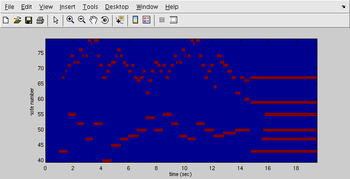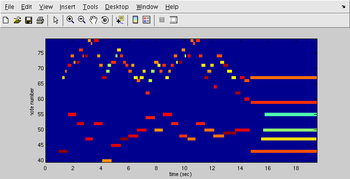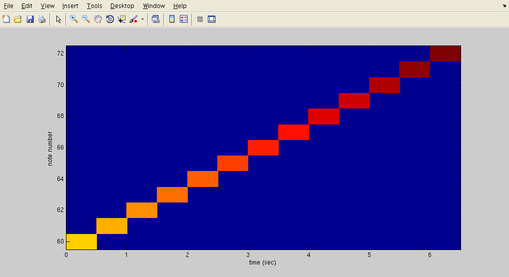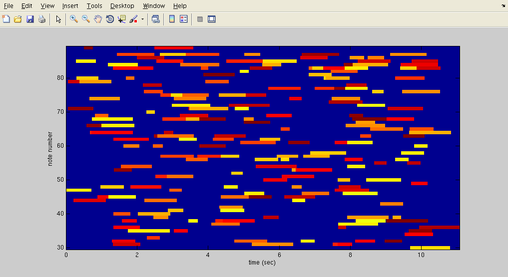# KenSchutte.com

## MATLAB and MIDI

I've written some MATLAB / GNU Octave scrips to read and write MIDI files.

Included are some very basic synthesis functions, which can easily be replaced by your own code. MIDI can be also be generated from note information you create within matlab: perhaps useful for audio transcription or algorithmic composition.

Here's an example of reading in the included file, jesu.mid. First, readmidi.m parses the file into messages and stores them in a matlab struct.

```>> midi = readmidi('jesu.mid')

midi =

format: 0
ticks_per_quarter_note: 384
track: [1x1 struct]
```

`midi2audio` will synthesize this midi information to a waveform. The default uses a simple FM synthesis technique. This can then be written to a file, etc.

```% synthesize with FM-synthesis.
% (y = audio samples.  Fs = sample rate.  Here, uses default 44.1k.)
[y,Fs] = midi2audio(midi);

%% listen in matlab:
soundsc(y, Fs);  % FM-synth

% There are two other very basic synth methods included:
y = midi2audio(midi, Fs, 'sine');
soundsc(y,Fs);

y = midi2audio(midi, Fs, 'saw');
soundsc(y,Fs);

% save to file:
% (normalize so as not clipped in writing to wav)
y = .95.*y./max(abs(y));
wavwrite(y, Fs, 'out.wav');
```

To add or modify synthesis algorithms, you can change or replace the 'synth.m' file.

### Analyzing the MIDI file

Internally, midi2audio uses the midiInfo function to generate start and end times of all notes in the file. midiInfo will display a list of the midi messages in the file:

```>> midi = readmidi('jesu.mid');>>
midiInfo(midi);
--------------------------------------------------
Track 1
--------------------------------------------------

-     0        0:0.000      meta     Set Tempo          microsec per quarter note: 500000
-     0        0:0.000      meta     Time Signature     4/2, clock ticks and notated 32nd notes=24/8
0     751      0:0.978               Note on            nn=43  vel=99
0     171      0:1.201               Note on            nn=67  vel=68
0     133      0:1.374               Note on            nn=67  vel=0
0     29       0:1.411               Note on            nn=69  vel=75
0     91       0:1.530               Note on            nn=69  vel=0
.
.
.
```

midiInfo also returns a matrix of size Nx8, where N is the number of notes in the file. The 8 colunms indicate:

1. track number
2. channel number
3. note number (midi encoding of pitch)
4. velocity
5. start time (seconds)
6. end time (seconds)
7. message number of note_on
8. message number of note_off
```>> midi = readmidi('jesu.mid');>>
Notes = midiInfo(midi,0);>>
whos Notes
Name        Size                    Bytes  Class

Notes      90x8                      5760  double array

Grand total is 720 elements using 5760 bytes

>> Notes(1:5,:)

ans =

1.0000         0   43.0000   99.0000    0.9779    1.6120    3.0000    8.0000
1.0000         0   67.0000   68.0000    1.2005    1.3737    4.0000    5.0000
1.0000         0   69.0000   75.0000    1.4115    1.5299    6.0000    7.0000
1.0000         0   71.0000   88.0000    1.6250    1.8268    9.0000   12.0000
1.0000         0   55.0000   88.0000    1.6354    2.1979   10.0000   16.0000
```

### Piano Roll

This "Notes" matrix can easily be used to create a "piano-roll" display. I've included a script for this:

```%% compute piano-roll:
[PR,t,nn] = piano_roll(Notes);

%% display piano-roll:
figure;
imagesc(t,nn,PR);
axis xy;
xlabel('time (sec)');
ylabel('note number');

%% also, can do piano-roll showing velocity:
[PR,t,nn] = piano_roll(Notes,1);

figure;
imagesc(t,nn,PR);
axis xy;
xlabel('time (sec)');
ylabel('note number');
```

This results in:### Writing MIDI

Simple scale

writemidi.m and matrix2midi.m allow you generate some notes somehow in Matlab, and write them out to a MIDI file. Here's a simple example of creating a chromatic scale:

```% initialize matrix:
N = 13;  % number of notes
M = zeros(N,6);

M(:,1) = 1;         % all in track 1
M(:,2) = 1;         % all in channel 1
M(:,3) = (60:72)';      % note numbers: one ocatave starting at middle C (60)
M(:,4) = round(linspace(80,120,N))';  % lets have volume ramp up 80->120
M(:,5) = (.5:.5:6.5)';  % note on:  notes start every .5 seconds
M(:,6) = M(:,5) + .5;   % note off: each note has duration .5 seconds

midi_new = matrix2midi(M);
writemidi(midi_new, 'testout.mid');
```

Note, you only have to specify the first 6 columns of the note matrix (7 and 8 hold the index of MIDI messages). Now, you should have a valid MIDI file to open elsewhere. If you're on Linux, you might have timidity installed,

```\$ timidity testout.mid
# hopefully you hear something...

# or convert to wave, and play with wave player:
\$ timidity -o testout.wav -Ow testout.mid
\$ aplay testout.wav
```

Random Notes

Now, let's try creating some random music: let's say 200 random notes with random start times and volumes, etc...

```% initialize matrix:
N = 200;
M = zeros(N,6);

M(:,1) = 1;         % all in track 1
M(:,2) = 1;         % all in channel 1
M(:,3) = 30 + round(60*rand(N,1));  % random note numbers
M(:,4) = 60 + round(40*rand(N,1));  % random volumes
M(:,5) = 10 * rand(N,1);
M(:,6) = M(:,5) + .2 + rand(N,1);  % random duration .2 -> 1.2 seconds

midi_new = matrix2midi(M);
writemidi(midi_new, 'testout2.mid');
```

This sounds much cooler with timidity's grand piano synth:

```\$ timidity testout2.mid
```

Congratulations! You're now a modern art composer.

These result in the following piano-rolls:### Notes

• `readmidi` should be able to read any MIDI file - it does little parsing except breaking up into individual messages (handles deltatimes, running-mode, and determines the message type).
• In synthesis, many message types are ignored (for example, it doesn't handle controller messages, e.g. pitch-wheel bends, etc.).
• Using readmidi and writemidi with no modifications can be used to test behavior. For example, run something like,
```>> writemidi(readmidi('orig.mid'),'new.mid');
```
and check that 'orig.mid' and 'new.mid' are identical binary files. If you find this doesn't create an identical file, please let me know (but make sure it's a valid MIDI file). Or, you can do the check entire in Matlab, (since writemidi returns a byte string)
```>> midi = readmidi('orig.mid',1);    %% need to return rawbytes...>>
isequal(midi.rawbytes_all, writemidi(midi,'new.mid'))
```

### Status and Future

• I would be happy to hear about about any bugs and keep this up to date.
• However, I don't plan on making any major improvements to this. I no longer use Matlab at all. I've transitioned to Python (numpy, scipy, ipython, matplotlib) and will never look back!

### Files

The files are hosted on github. The project includes these files:

 readmidi.m reads MIDI file into a Matlab structure midi2audio.m convert the Matlab midi structure into an audio vector midiInfo.m display detailed information about MIDI Matlab structure synth.m synthesize a single note getTempoChanges.m finds times of all tempo changes midi2freq.m convert MIDI note number (0-127) to frequency in Hz matrix2midi.m create MIDI Matlab structure from a matrix with information on individual notes writemidi.m write a MIDI Matlab structure (such as created by readmidi) to a MIDI file

There is more documentation within each m-file. For example type, `help midi2audio` in MATLAB.